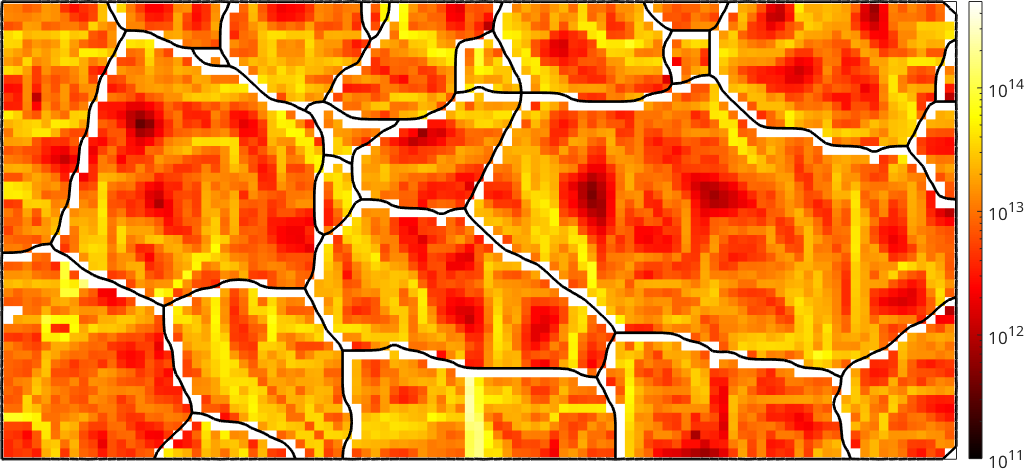Geometrically Necessary Dislocations edit page

This example sheet describes how to estimate dislocation densities following the reference paper

Pantleon, Resolving the geometrically necessary dislocation content by conventional electron backscattering diffraction, Scripta Materialia, 2008

Lets start by importing orientation data from 2 percent uniaxial deformed steel DC06 and visualize those data in an ipf map.In the next step we reconstruct grains, remove all grains with less then 5 pixels and smooth the grain boundaries.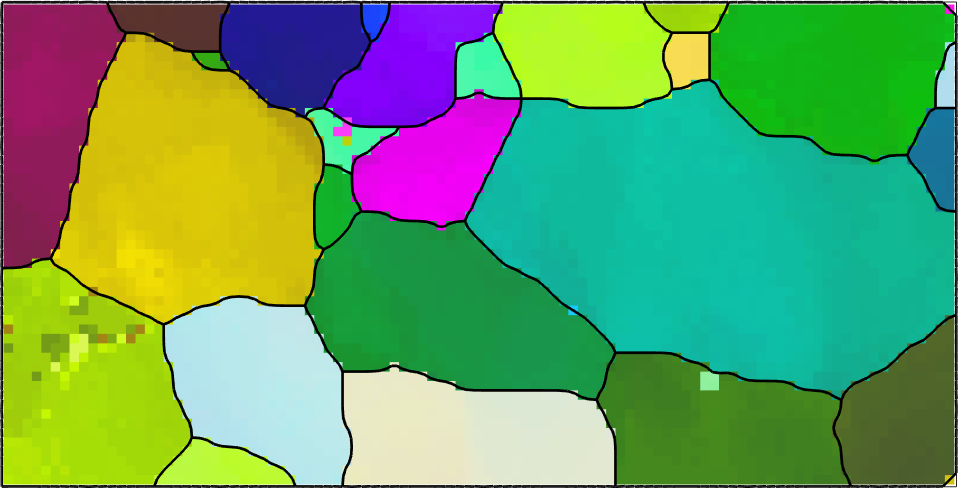## Data cleaning

The computation of geometrically neccesary dislocations from EBSD maps depends on local orientation changes in the map. In order to make those visible we switch to a different color key that colorises the misorientation of an pixel with respect to the grain meanorientation.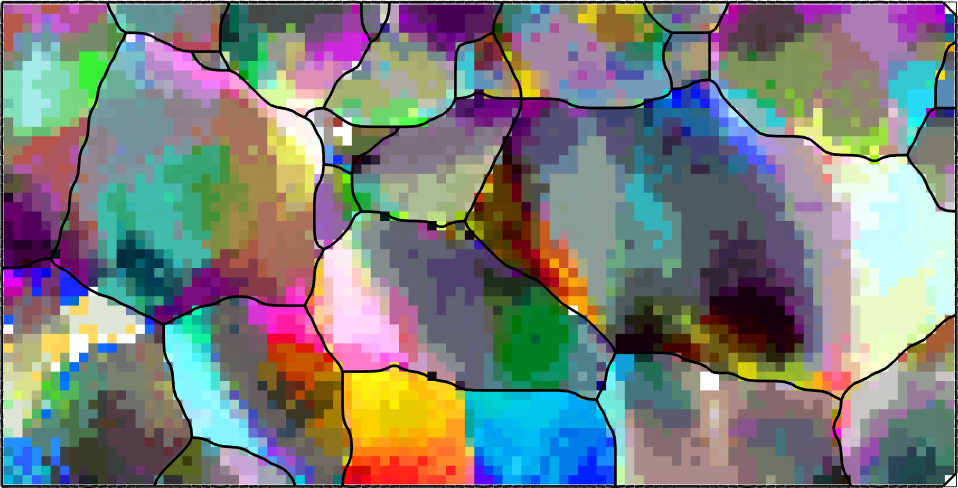We observe that the data are quite noisy. As noisy orientation data lead to overestimate the GND density we apply sime denoising techniques to the data.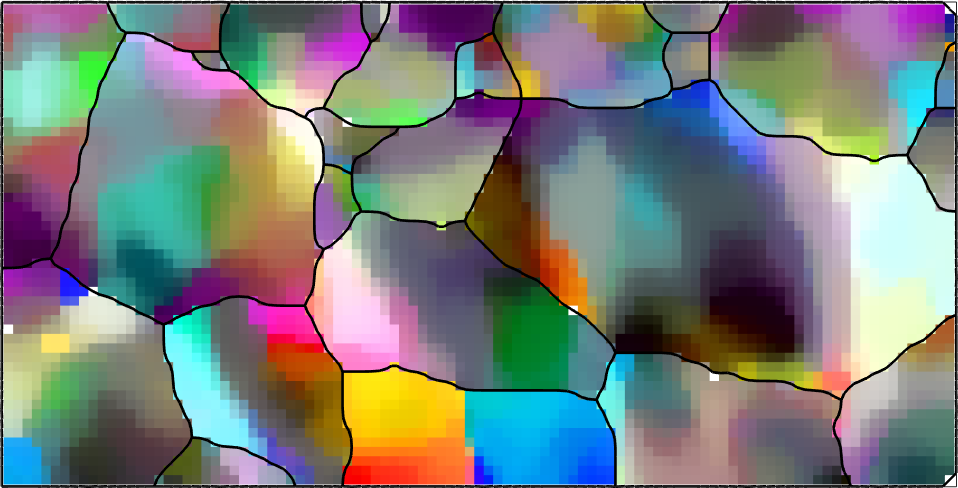## The incomplete curvature tensor

Starting point of any GND computation is the curvature tensor, which is a rank two tensor that is defined for every pixel in the EBSD map by the directional derivatives in x, y and z direction.

## The components of the curvature tensor

As expected the curvature tensor is NaN in the third column as this column corresponds to the directional derivative in z-direction which is usually unknown for 2d EBSD maps.

We can access the different components of the curvature tensor with

which results in a variable of the same size as our EBSD map. This allows us to visualize the different components of the curvature tensor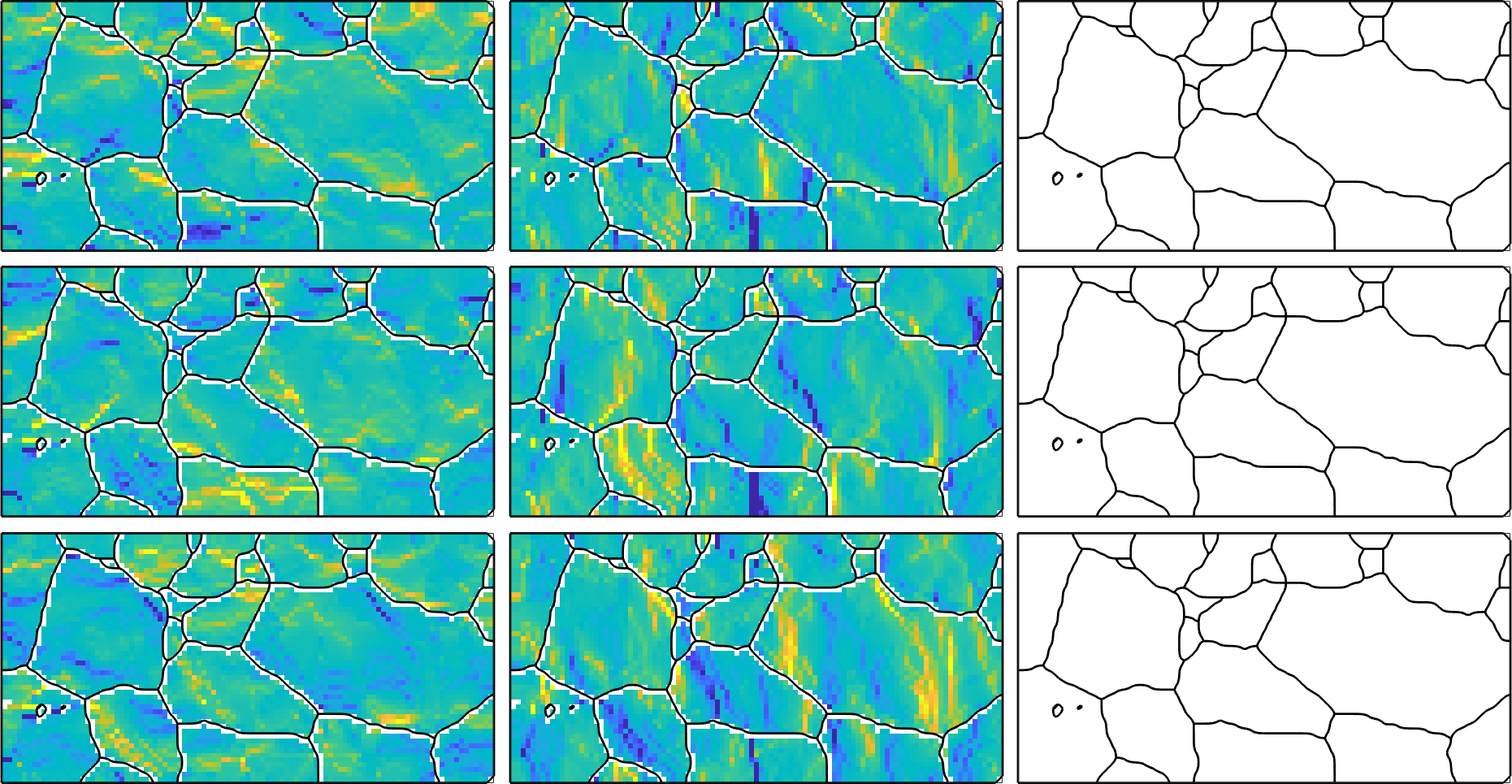## The incomplete dislocation density tensor

According to Kroener the curvature tensor is directly related to the dislocation density tensor.

which has the same unit as the curvature tensor and is incomplete as well as we can see when looking at a particular one.

## Crystallographic Dislocations

The central idea of Pantleon is that the dislocation density tensor is build up by single dislocations with different densities such that the total energy is minimum. Depending on the attomic lattice different dislocattion systems have to be considered. In present case of a body centered cubic (bcc) material 48 edge dislocations and 4 screw dislocations have to be considered. Those principle dislocations are defined in MTEX either by their Burgers and line vectors or by

Here the norm of the Burgers vectors is important

## The Energy of Dislocations

The energy of each dislocation system can be stored in the property u. By default this value it set to 1 but should be changed accoring to the specific model and the specific material.

According to Hull & Bacon the energy U of edge and screw dislocations is given by the formulae

$U_{\mathrm{screw}} = \frac{Gb^2}{4\pi} \ln \frac{R}{r_0}$

$U_{\mathrm{edge}} = (1-\nu) U_{\mathrm{screw}}$

where

• G is
• b is the length of the Burgers vector
• nu is the Poisson ratio
• R
• r

In this example we assume

R = r_0 = U = norm(dS.b).^2

A single dislocation causes a deformation that can be represented by the rank one tensor

Note that the unit of this tensors is the same as the unit used for describing the length of the unit cell, which is in most cases Angstrom (au). Furthremore, we observe that the tensor is given with respect to the crystal reference frame while the dislocation densitiy tensors are given with respect to the specimen reference frame. Hence, to make them compatible we have to rotate the dislocation tensors into the specimen reference frame as well. This is done by

## Fitting Dislocations to the incomplete dislocation density tensor

Now we are ready for fitting the dislocation tensors to the dislocation densitiy tensor in each pixel of the map. This is done by the command fitDislocationSystems.

As result we obtain a matrix of densities rho such that the product with the dislocation systems yields the incomplete dislocation density tensors derived from the curvature, i.e.,

we may also restore the complete curvature tensor with

and plot it as we did before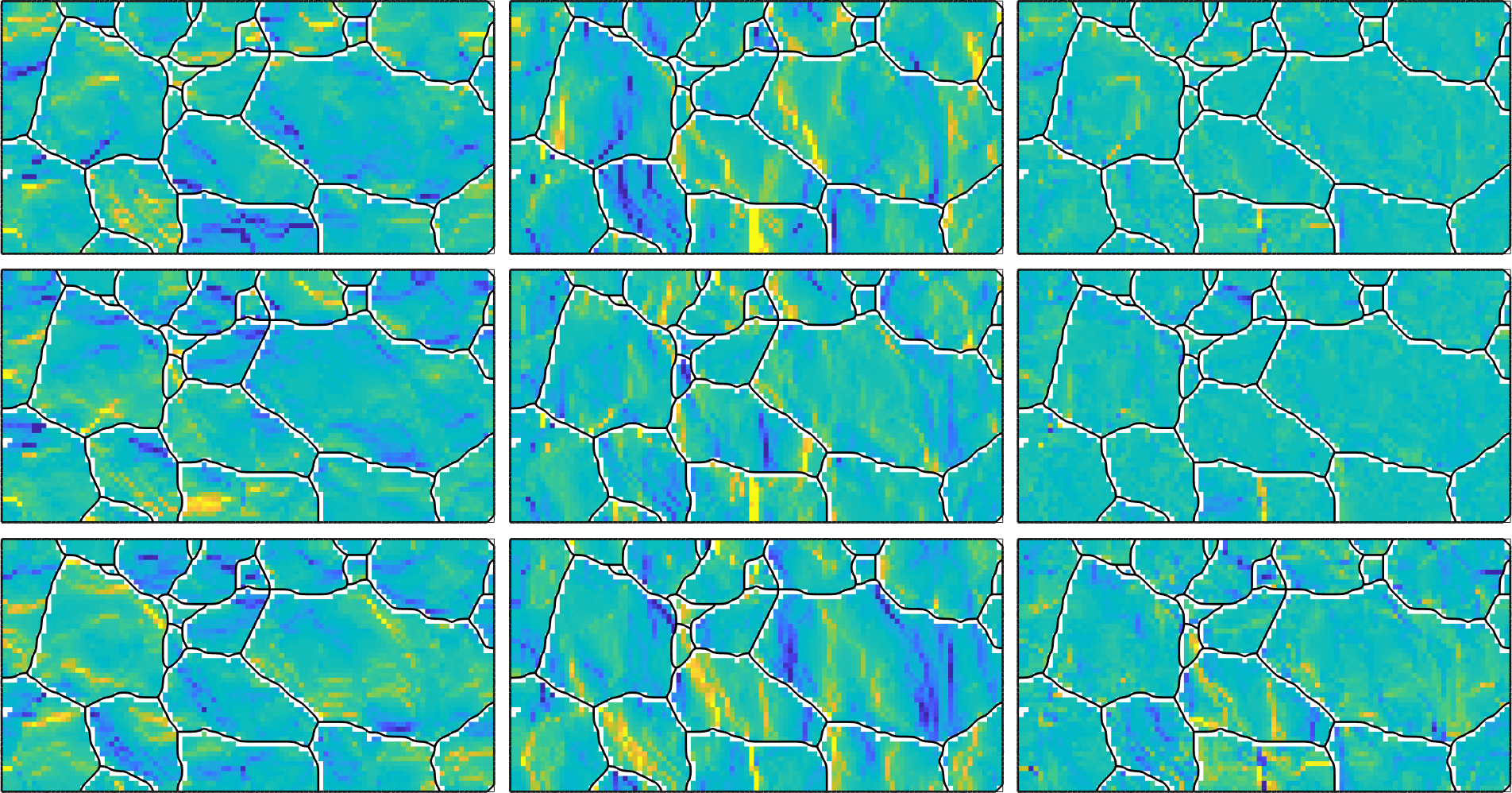## The total dislocation energy

The unit of the densities h in our example is 1/um * 1/au where 1/um comes from the unit of the curvature tensor an 1/au from the unit of the Burgers vector. In order to transform h to SI units, i.e., 1/m^2 we have to multiply it with 10^16. This is exactly the values returned as the second output factor by the function fitDislocationSystems.

Multiplying the densities rho with this factor and the individual energies of the the dislocation systems we end up with the total dislocation energy. Lets plot this at a logarithmic scale